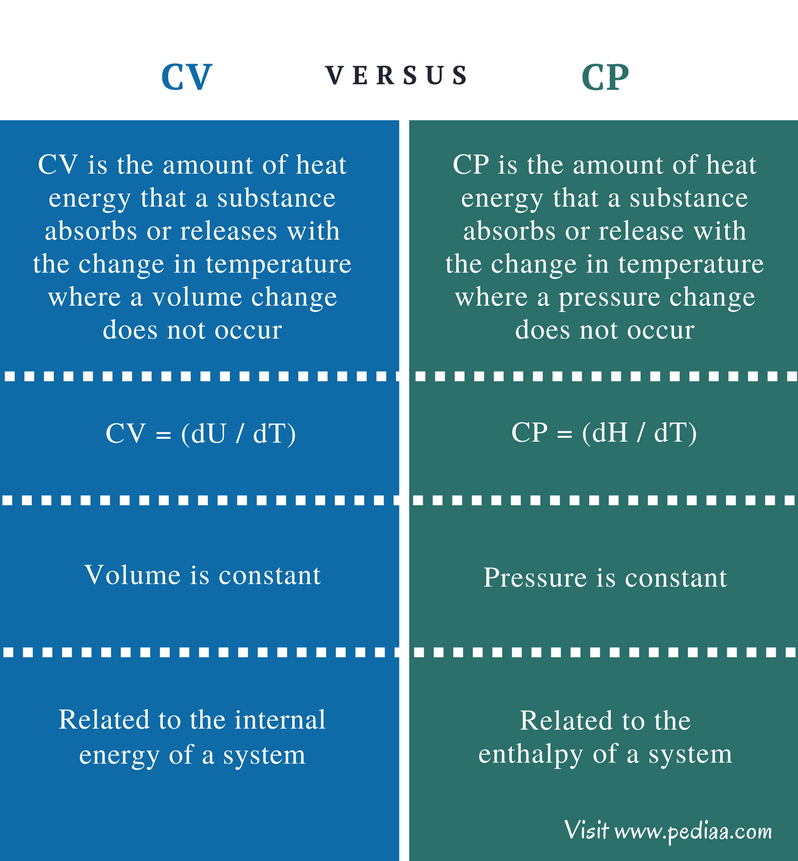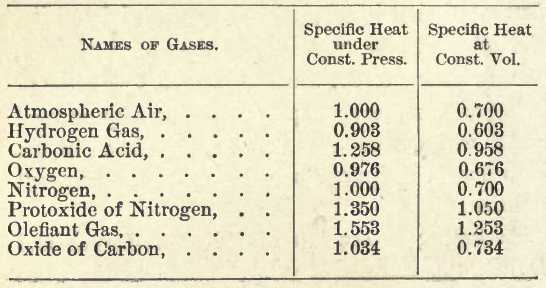# Difference Between CV and CP

## Main Difference – CV vs CP

Thermodynamics is a branch of physical chemistry that describes the relationship of heat energy with other forms of energy. CV and CP are two terms used in thermodynamics. CV is the specific heat at constant volume, and CP is the specific heat at constant pressure. Specific heat is the heat energy required to raise the temperature of a substance (per unit mass) by one degree Celsius. The main difference between CV and CP is that the volume change is zero for a system under CV whereas the pressure change is zero for a system under CP.

### Key Areas Covered

1. What is CV
– Definition, Properties, Formula
2. What is CP
– Definition, Properties, Formula
3. What is the Relationship Between CV and CP
– Outline of Common Features
4. What is the Difference Between CV and CP
– Comparison of Key Differences

Key Terms: CP, CV, Specific Heat, Pressure, Thermodynamics, Volume## What is CV

CV is the amount of heat energy that a substance absorbs or releases (per unit mass) with the change in temperature where a volume change does not occur. In other words, it is the heat energy transferred between a system and its surrounding without any change in the volume of the system.

This term is related to the internal energy of a system. The internal energy is the sum of potential and kinetic energy of a system. When the temperature of that system is changed, the system may absorb energy from outside or release energy to the surrounding. If this change of internal energy occurs at a constant volume, then it is the specific heat at constant volume, CV. This can be symbolized as below.Where,

CV is the specific heat at constant volume

dU is the change in internal energy

dT is the change in temperatureFigure 01: A table of specific heats of some gases.

The above image shows a table of specific heats of some common gases. The specific heat at constant pressure and constant volume are given in the units of cal/g/oC.

## What is CP

CP is the specific heat at constant pressure. It is the amount of energy released or absorbed by a unit mass of substance with the change in temperature at constant pressure. In other words, it is the energy transferred between a system and its surrounding under constant pressure.

This term is related to the enthalpy of a system. Enthalpy is the energy absorbed or released. It is defined as the sum of the internal energy and the product of pressure and volume. That is because the total energy that a system absorbs or releases is equal to the amount of energy that the system already has (internal energy) and changes that occur in the system (PV). The change in temperature will cause a change in enthalpy of the system. Therefore, this can be given as below.Where,

CP is the specific heat at constant pressure

dH is the enthalpy change

dT is the change in temperature

## Relationship Between CV and CP

• The following relationship can be given considering the ideal gas behavior of a substance.

CP – CV = R

Where r is the universal gas constant.

• The ratio between CP and CV is the specific heat ratio, γ.

Γ = CP /CV

## Difference Between CV and CP

### Definition

CV: CV is the amount of heat energy that a substance absorbs or releases (per unit mass) with the change in temperature where a volume change does not occur.

CP: CP is the amount of heat energy that a substance absorbs or release (per unit mass) with the change in temperature where a pressure change does not occur.

### Constant Parameters

CV: In CV, volume is constant.

CP: In CP, pressure is constant.

### Related Parameters

CV: CV is related to the internal energy of a system.

CP: CP is related to the enthalpy of a system.

### Conclusion

Specific heats are thermodynamic properties of substances. These properties explain the behavior of a substance or a system with the changes of temperature of that system. The concept of specific heat is true for ideal gases, but it often has to be modified when using with normal gases. This is because the behavior of gases typically depends on both temperature and the pressure.

##### References:

1.”The relation between temperature change and heat.” 2.4 Specific Heats. N.p., n.d. Web. Available here.  18 July 2017.
2.”Heat capacity.” Wikipedia. Wikimedia Foundation, 13 July 2017. Web. Available here. 18 July 2017.

##### Image Courtesy:

1. “Specific heat table” By William A. Kelley – Own work (CC BY-SA 4.0) via Commons Wikimedia